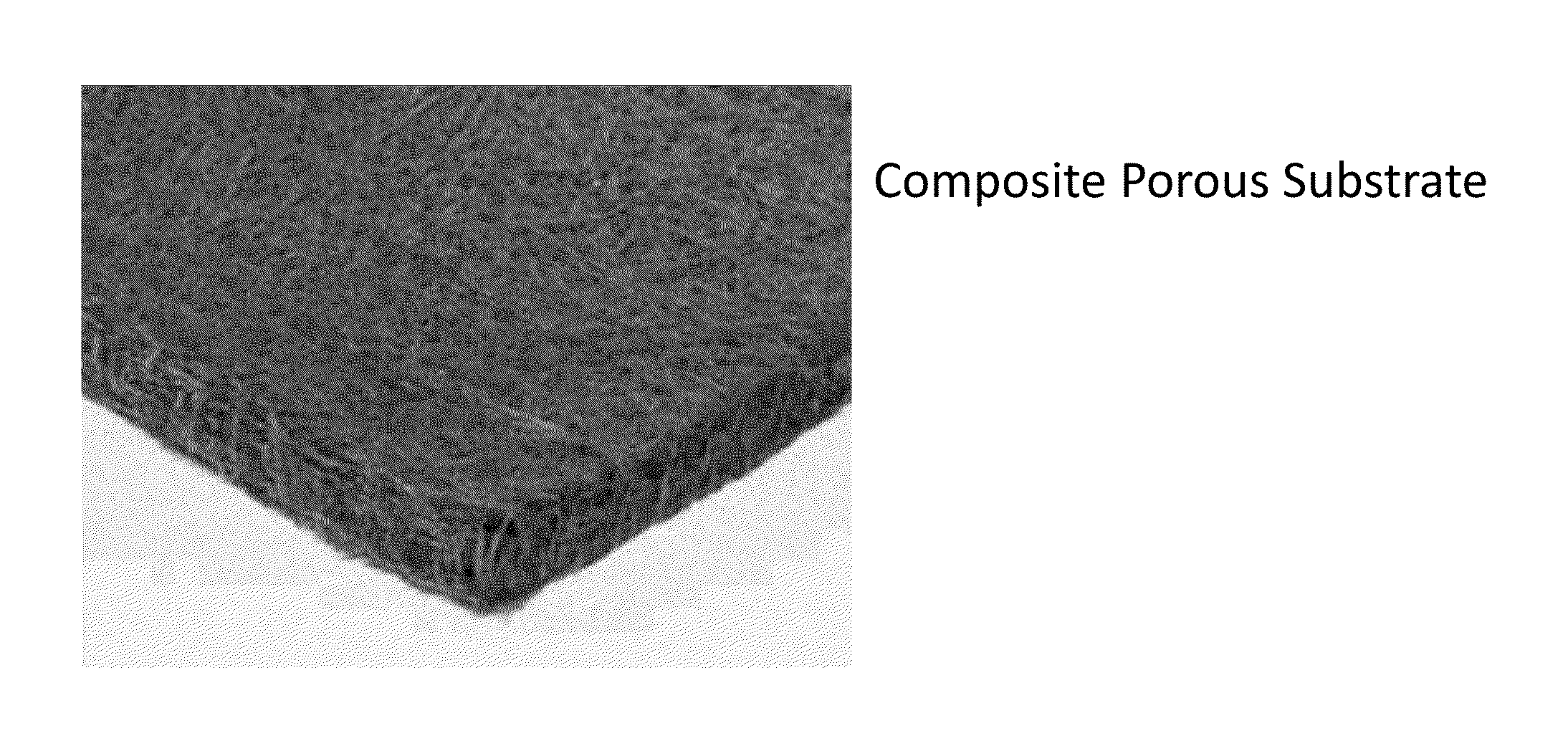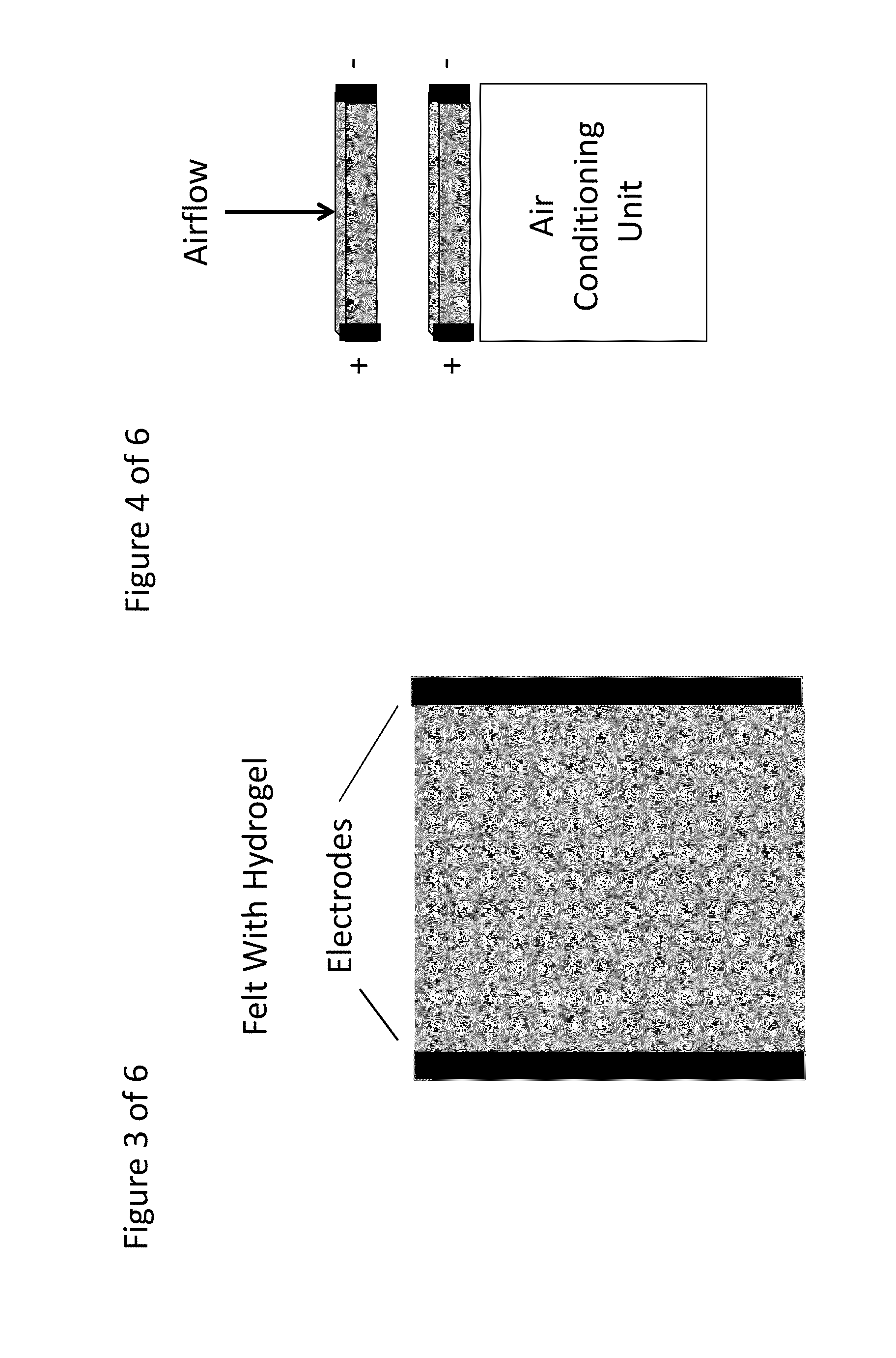# Composite Porous Fibrous Dehumidifying Material

Inactive Publication Date: 2013-11-21
WORLD ENVIRONMENTAL SOLUTIONS
6 Cites 30 Cited by

## AI-Extracted Technical Summary

### Problems solved by technology

The pressure exerted by the vapor in the air is higher, so the vapor molecules move from the air to the desiccant, which is typically a layered, hydrophilic alumina silicate matrix that can tie up “liquid” phase water, resulting in dehumidifying the air.
Whil...
View more

### Benefits of technology

In a preferred embodiment the external stimulus is selected from a temperature change, pH ...
View more

## Abstract

A composite porous fibrous dehumidifying material comprised of a plurality of fibers whose surface has immobilized thereon a reversible hydrogel material. The composite porous fibrous structure can be used as a dehumidifying material for reducing energy consumption in air conditioning/climate control units. Preferred fibrous materials are electrically conductive materials, such a graphitic fibers.

Application Domain

Lighting and heating apparatusSynthetic resin layered products +3

Technology Topic

Electrically conductiveConductive materials +4

## Image

•••## Examples

• Experimental program(3)

### Example

Example 1
Comparative
System: Vapor Compression—
The most commonly used air conditioning systems:
Qtotal=Qlatent+Qsensible
TABLE I 90% RH 55% RH Latent Heat Energy Q latent to be removed ( BTU ) = Mass of Air × ( Q latent ( in ) - Q latent ( out ) ( In - Out ) grains lb . of Dry air × 1 lb water 7000 grains × 100 lbs . of Dry air × 1000 BTUs lb . of water evaporated ((120 − 54)/7000) × 100 × 1000 = (70 − 54/7000) × 100 × 1000 = 943 BTUs 229 BTUs of latent heat to be removed of latent heat to be removed Sensible Heat Energy To Take Dry Air From 75° F. to 50° F. Qsensible to be removed (BTU) = Mass of Air (lbs.) × (Heat Capacity(in) − Heat Capacity(out)) BTUs/lb. of air ×ΔT (° F.) 100 lbs. × (0.24) BTUs/lb.-° F. × 100 lbs. × (0.24) BTUs/lb.-° F. × 25° F. = 25° F. = 600 BTUs 600 BTUs of sensible heat to be removed of sensible heat to be removed
Typical Coefficient Of Performance for vapor compression systems is 3.0, if operating at a coil temperature of 45° F.
TABLE II 90% RH 55% RH Energy required to remove heat load Sensible Heat + latent Heat Divided by Coefficient of Performance ( 600 + 943 ) / 3 = 880 BTUs = 514 BTUs × 1 KW - hr 3413 BTUs = 0.151 KW - hr ( 600 + 229 ) / 3 = 643 BTUs = 643 BTUs × 1 KW - hr 3413 BTUs = 0.181 KW - hr

### Example

Example 2
Comparative
System: Vapor Compression Air Conditioner Using Desiccant Wheel Dehumidifier—
System based on zeolites (such as hydrophilic alumina silicates, typically used as desiccants).
In this system, the latent heat from the phase change of the water vapor to liquid phase is passed on to the dehumidified air, raising its temperature.  1. Exit air temperature from the desiccant=94.2° F.  2. Water Vapor content of exit air=54 grains/lb. of air.  3. Specific Heat Capacity of desiccant=0.22 BTU/lb.-° F.  4. Specific Heat Capacity of air=0.24 BTU/lb.-° F.  5. Heat carryover=latent heat of vaporization of water.  6. Airstream cooled back to input airstream temperature (75° F.), which will require removal of latent heat that was transferred by the desiccant to the dehumidified air.  7. Coil temperature for the vapor compression based cooling system will be at 60° F.
TABLE III 90% RH 55% RH No Latent Heat Energy Required As Air Will be Dried By Dessicant Sensible Heat Energy To Take Dry Air From Higher Dry air Temperature will be the amount of latent heat in each RH condition plus the sensible heat calculated before for a 75° F. to 50° F. temperature drop Qsensible to be removed (BTU) = [Mass of Air (lbs.) × (Heat Capacity(air)) BTUs/lb. of air ×ΔT (° F.)] + Latent Heat generated by Dessicant and carried over to the air 100 lbs. × (0.24) BTUs/lb.-° F. × 100 lbs. × (0.24) BTUs/lb.-° F. × 25° F. = 600 + 943 (Latent Heat) = 25° F. = 600 + 229 (Latent Heat) = 1,400 BTUs 830 BTUs of sensible heat to be removed of sensible heat to be removed
Typical Coefficient Of Performance for vapor compression systems is 4.0, if operating at a coil temperature of 60° F.
TABLE IV 90% RH 55% RH Energy required to remove heat load Sensible Heat + latent Heat Divided by Coefficient of Performance 1400 / 4 = 350 BTUs = 350 BTUs × 1 KW - hr 3413 BTUs = 0.103 KW - hr 830 / 4 = 208 BTUs = 208 BTUs × 1 KW - hr 3413 BTUs = 0.061 KW - hr
In this system, one must consider the energy required to regenerate the desiccant. Very few installations have available waste heat at such high temperatures.  Desiccant regeneration energy (theoretical) required would be the latent heat required for the absorbed water to be vaporized.  Energy conversion efficiency of energy source to heat is taken at 90%.
TABLE V 90% RH 55% RH Energy required to evaporate water from dessicant 943/0.9 = 1,048 BTUth 229/0.9 = 255 BTUth

### Example

Example 3
System: Vapor Compression Air Conditioner Using Rapid Kinetics Reversible Hydrogel of Present Invention.  Input and output requirements assumed to be same.  Gel absorbs 50% of its weight in water.  2.0 lbs. of gel needed per lb. of water.  Gel Specific Heat Capacity=0.6 BTU/lb.-° F. (measured data)  Gel temperature rise per lb. of water=9° F. (measured)  The carryover heat in this case will be the slight exothermic nature of gel “expansion” when the water vapor deliquesces it.  Cooling by conventional vapor compression remains the same with a COP of 4.0 at a coil temperature of 60° F.
TABLE VI 90% RH 55% RH No Latent Heat Energy Required As Air Will be Dried By Gel We will need to provide the energy to cool the air from the inlet to outlet condition, and also provide the energy to cool the slight amount of heat generated due to an exothermic nature of gel expansion. Q sensible to be removed ( BTU ) = [ Mass air lbs × Heat Capacity ( air ) BTUs / lb air × ΔT air ( ° F . ) ] + Mass of gel ( lbs . ) × Heat Capacity of Gel ( BTU / lb gel - ° F . ) × ΔT gel ° F . ( Heat generated by Gel and carried over to the air ) = [ 100 lbs . × 0.24 BTUs / lb air × 25 ( ° F . ) ] + 2 ( lbs . ) × 0.6 BTU / lb gel - ° F . × 9 ° F . = 612 BTUs Electrical Energy = 612 / 4 = 153 BTUs × 1 KW - hr 3413 BTUs = 0.045 KW - hrs Virtually same in all realistic RH conditions
CYCLE LENGTH CALCUATION : _ Capacity gel = 0.5 lbs water Humidity removed under 90 % RH conditions Per 100 lbs . of AIR : ( 120 - 54 ) grains × 1 lb . 7000 grains = 0.00943 lbs . 0.5 lbs water ÷ 0.00943 lbs per cycle = 53 times the base amount of 100 lb of air used in this example . Thus the energy consumption is divided by this number for a per 100 lb air calculation . Regeneration of the 2 lb . of gel takes 20 minutes ( 1 / 3 hr ) of 2 amps × 220 V current . Total energy consumed = 440 watts / 3 = 113 watt hour . = 113 watt - hrs / 53 cycles = 0.002 KW - hrs for the example above . Total Energy consumed = 0.002 + 0.0045 KW - hrs = 0.047 KW - hrs .

## PUM

 Property Measurement Unit Temperature 25.0 °C Temperature 100.0 °C Fraction 0.001 ~ 0.5 fraction

## Description & Claims & Application Information

We can also present the details of the Description, Claims and Application information to help users get a comprehensive understanding of the technical details of the patent, such as background art, summary of invention, brief description of drawings, description of embodiments, and other original content. On the other hand, users can also determine the specific scope of protection of the technology through the list of claims; as well as understand the changes in the life cycle of the technology with the presentation of the patent timeline. Login to view more.
Who we serve
• R&D Engineer
• R&D Manager
• IP Professional
Why Eureka